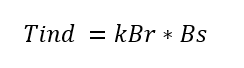# Why Synchronous Motor is Not Self Starting [Simple Explanation]

### Synchronous Motor is Not Self Starting:

Why synchronous motor is not self-starting? Do you know the real reason? Do know, actually the net torque developed in the motor is zero at starting of the motor, that’s why the motor cannot rotate the rotor shaft at the time of starting. Let see why the torque is zero.

For simple understanding, we take a single electrical cycle of 50Hz. Here, Bs is the magnetic field developed in the stator and it is rotating magnetic field. Br is the magnetic field developed in the rotor and it is a steady magnetic field. Tind is the Torque developed in the motor.

Let we consider complete cycle with a different instance.

#### At t=0:

Refer the picture 1.2, at t=0, the stator magnetic field Bs and rotor magnetic field Br is alleged with each other. They are magnetically locked.

The torque developer in the AC machine isHence the net torque produced in the motor is zero Tst=0

#### At t=1/200 s:

Refer to fig 1.1 and 1.3 At this time t=1/200 s, the rotor slightly moves but the stator magnetic field now points to the left. By the induced-torque equation, the torque produced in the rotor will be the counter-clockwise direction.

#### At t=1/100 s:

At this instance (t=1/100 s), the rotor magnetic field Br and stator magnetic field Bs pointing in opposite directions, and hence the torque produced in the rotor is zero.

#### t=3/200 s:

The stator magnetic field Bs now points to the right, and the resulting torque is clockwise. Hence the rotor shaft moves clockwise direction.